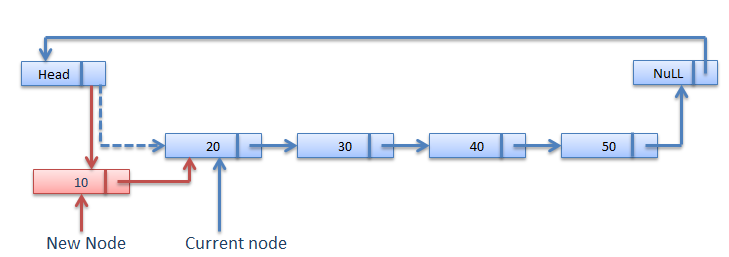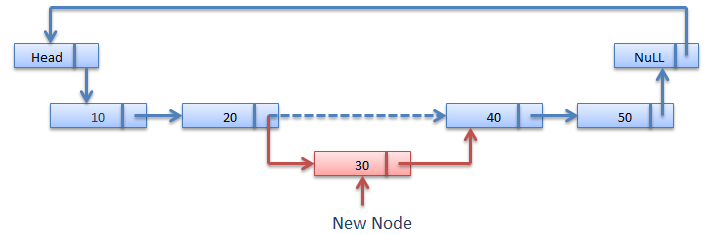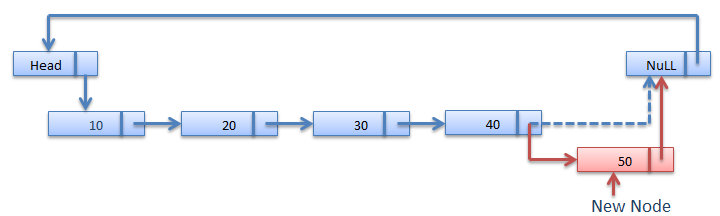Data Structure

# Insertion In Circular Linked List

There are three situation for inserting element in Circular linked list.
1.Insertion at the front of Circular linked list.
2.Insertion in the middle of the Circular linked list.
3.Insertion at the end of the Circular linked list.

## Insertion at the front of Circular linked list

### Procedure for insertion a node at the beginning of list

Step1. Create the new node
Step2. Set the new node’s next to itself (circular!)
Step3. If the list is empty,return new node.
Step4. Set our new node’s next to the front.
Step5. Set tail’s next to our new node.
Step6. Return the end of the list.### Algorithm for Insertion at the front of Circular linked list

```node* AddFront(node* tail, int num)
{
node *temp = (node*)malloc(sizeof(node));
temp->data = num;
temp->next = temp;
if (tail == NULL)
return temp;
temp->next = tail->next;
tail->next = temp;
return tail;
}
```

### Insertion in the middle of the Circular linked list

```InsertAtlocDll(info,next,start,end,loc,size)
1.set nloc = loc-1 , n=1
2.create a new node and address in assigned to ptr.
3.check[overflow] if(ptr=NULL)
write:overflow and exit
4.set Info[ptr]=item;
5.if(start=NULL)
set next[ptr] = NULL
set start = ptr
else if(nloc<=size)
repeat steps a and b while(n != nloc)

a.	loc = next[loc]
b.	n = n+1
[end while]
next[ptr] = next[loc]
next[loc] = ptr
else
set last = start;
repeat step (a) while(next[last]!= NULL)
a. last=next[last]
[end while]
last->next = ptr ;
[end if]
6.Exit.
```## Insertion at the end of Circular linked list

### Procedure for insertion a node at the end of list

Step1. Create the new node
Step2. Set the new node’s next to itself (circular!)
Step3. If the list is empty,return new node.
Step4. Set our new node’s next to the front.
Step5. Set tail’s next to our new node.
Step6. Return the end of the list.### Algorithm for Insertion at the End of Circular linked list

```node* AddEnd(node* tail, int num)
{
node *temp = (node*)malloc(sizeof(node));
temp->data = num;
temp->next = temp;
if (tail == NULL)
return temp;
temp->next = tail->next;
tail->next = temp;
return temp;
}
```